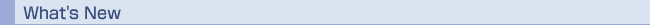超ロバスト計算原理プロジェクト『最適化・制御セミナー』 日時：平成１８年９月１９日（火）　15:00〜17:00 場所：工学部６号館セミナー室C (6号館372号室) 講演者：Dr. Fabio Tardella　(University of Rome ‘La Sapienza’) タイトル：Submodular function minimization in Zn and searching in Monge　arrays 概要 The theory and applications of submodular function minimization on (a sublattice of) the 0-1 hypercube has been thoroughly investigated in the last three decades. However, submodularity does appear and is usefully exploited in more general settings, possibly with different names. We consider the problem of minimizing a submodular function on a sublattice of Zn and we establish and use connections among this problem, the problem of searching in a multidimensional Monge array (arising in Computer Science and Computational Geometry), and submodular pseudo-Boolean function minimization. In particular, we obtain the first algorithm for minimizing a submodular function on a sublattice S of Zn a with time complexity polynomial in the length of a maximal chain of S, and we use it to construct a polynomial algorithm (regarded as a "major improvement'' by Aggarwal and Park) for the problem of searching in a multidimensional Monge array. We then show that submodular function minimization in Zn can be applied to solve efficiently some linear and nonlinear IPs that include Hochbaum's "monotone" IPs with two or three variables per inequality, and can be used to establish polinomiality for the problem of minimizing a linear function on (extensions of) Fujishige's Ternary Submodular Polyhedra. Joint work with Maurice Queyranne.